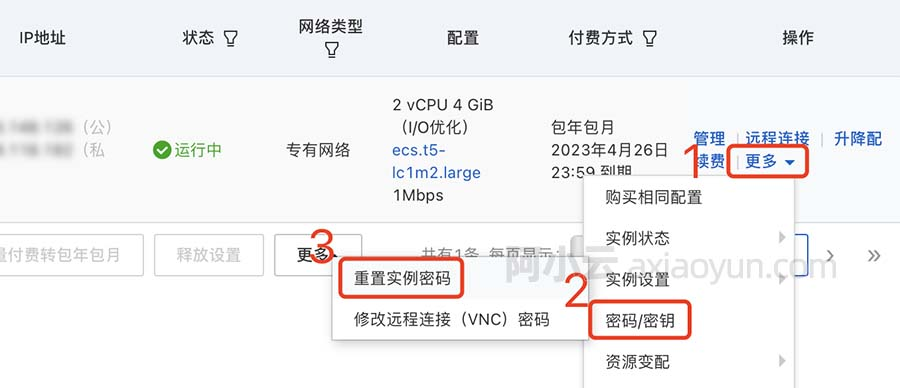# Spring JDBC框架

+关注继续查看

Spring JDBC提供了一些方法和相应不同的类与数据库进行交互。我要采取经典和最流行的做法，利用JdbcTemplateclass框架。这是管理的所有数据库的通信和异常处理中心框架类。

JdbcTemplate 类

JdbcTemplate类执行SQL查询，更新语句和存储过程调用，在结果集和提取返回参数值进行迭代。它还捕捉JDBC的异常并将其转换为通用的，信息更丰富，除了在org.springframework.dao包中定义的层次结构。

JdbcTemplate类的实例是一次配置的线程。所以，你可以配置一个JdbcTemplate的一个实例，然后安全地注入这种共享引用到多个DAO。

 CREATE TABLE Student( ID INT NOT NULL AUTO_INCREMENT, NAME VARCHAR(20) NOT NULL, AGE INT NOT NULL, PRIMARY KEY (ID) );

<bean id="dataSource" class="org.springframework.jdbc.datasource.DriverManagerDataSource">
<property name="driverClassName" value="com.mysql.jdbc.Driver"/>
<property name="url" value="jdbc:mysql://localhost:3306/TEST"/>
</bean>

DAO表示这是通常用于数据库交互的数据访问对象。 DAO的存在是为了提供读取和写入数据到数据库中，他们应该通过该应用程序的其余部分将访问它们的接口公开此功能的一种手段。

String SQL = "select count(*) from Student";
int rowCount = jdbcTemplateObject.queryForInt( SQL );

String SQL = "select count(*) from Student";
long rowCount = jdbcTemplateObject.queryForLong( SQL );

String SQL = "select age from Student where id = ?";
int age = jdbcTemplateObject.queryForInt(SQL, new Object[]{10});

String SQL = "select name from Student where id = ?";
String name = jdbcTemplateObject.queryForObject(SQL, new Object[]{10}, String.class);

String SQL = "select * from Student where id = ?";
Student student = jdbcTemplateObject.queryForObject(SQL, new Object[]{10}, new StudentMapper()); public class StudentMapper implements RowMapper<Student> { public Student mapRow(ResultSet rs, int rowNum) throws SQLException { Student student = new Student(); student.setID(rs.getInt("id")); student.setName(rs.getString("name")); student.setAge(rs.getInt("age")); return student; } }

String SQL = "select * from Student";
List<Student> students = jdbcTemplateObject.query(SQL, new StudentMapper()); public class StudentMapper implements RowMapper<Student> { public Student mapRow(ResultSet rs, int rowNum) throws SQLException { Student student = new Student(); student.setID(rs.getInt("id")); student.setName(rs.getString("name")); student.setAge(rs.getInt("age")); return student; } }

String SQL = "insert into Student (name, age) values (?, ?)";
jdbcTemplateObject.update( SQL, new Object[]{"Zara", 11} );

String SQL = "update Student set name = ? where id = ?";
jdbcTemplateObject.update( SQL, new Object[]{"Zara", 10} );

String SQL = "delete Student where id = ?";
jdbcTemplateObject.update( SQL, new Object[]{20} );

String SQL = "CREATE TABLE Student( " + "ID INT NOT NULL AUTO_INCREME64001 015967 0Xshell使用SSH远程登录阿里云ECS服务器CentOS7
22365 023590 022367 019357 016672 022544 019825 0windows server 2008阿里云ECS服务器安全设置

11998 0

7195

9

JS零基础入门教程（上册）